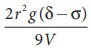Home | | Physics 11th std | Viscosity of a Liquid By StokeŌĆÖs Method

# Viscosity of a Liquid By StokeŌĆÖs Method

To determine the co-efficient of viscosity of the given liquid by stokeŌĆÖs method

VISCOSITY OF A LIQUID BY STOKEŌĆÖS METHOD

## AIM

To determine the co-efficient of viscosity of the given liquid by stokeŌĆÖs method

## APPARATUS REQUIRED

A long cylindrical glass jar, highly viscous liquid, metre scale, spherical ball, stop clock, thread.

## FORMULAWhere

╬Ę - Coefficient of viscosity of liquid (N s mŌĆō2)

r ŌåÆ radius of spherical ball ( m )

╬┤ŌåÆ density of the steel sphere ( kg mŌĆō3 )

ŽāŌåÆ density of the liquid ( kg mŌĆō3 )

g ŌåÆ acceleration due to gravity (9.8 m sŌĆō2 )

V ŌåÆ mean terminal velocity ( m sŌĆō1 )

## DIAGRAM## PROCEDURE

┬Ę         A long cylindrical glass jar with markings is taken.

┬Ę         Fill the glass jar with the given experimental liquid.

┬Ę         Two points A and B are marked on the jar. The mark A is made well below the surface of the liquid so that when the ball reaches A it would have acquired terminal velocity V.

┬Ę         The radius of the metal spherical ball is determined using screw gauge.

┬Ę         The spherical ball is dropped gently into the liquid.

┬Ę         Start the stop clock when the ball crosses the point A. Stop the clock when the ball reaches B.

┬Ę         Note the distance between A and B and use it to calculate terminal velocity.

┬Ę         Now repeat the experiment for different distances between A and B. Make sure that the point A is below the terminal stage.

## OBSERVATIONS

To find Terminal Velocity:## CALCULATION

Density of the spherical ball ╬┤ = ________ kg mŌłÆ3

Density of the given liquid Žā = ________ kg mŌłÆ3

Coefficient of viscosity of the liquid ╬Ę = 2r2g(╬┤ ŌłÆŽā) / 9V  == ________ N s mŌĆō2

## RESULT

The coefficient of viscosity of the given liquid by stokeŌĆÖs method ╬Ę = ________ NsmŌĆō2

Tags : Physics Laboratory Practical Experiment , 11th Physics : Practical Experiment
Study Material, Lecturing Notes, Assignment, Reference, Wiki description explanation, brief detail
11th Physics : Practical Experiment : Viscosity of a Liquid By StokeŌĆÖs Method | Physics Laboratory Practical Experiment